# ResponsiveGridLayout

`ResponsiveGridLayout` is a container where the components are laid out on a grid, based on Bootstrap’s 12 columns grid system (see Bootstrap documentation for details).

Component’s XML-name: `responsiveGridLayout`.

The main feature of this container is that it is fully responsive. The arrangement of the components located inside the `ResponsiveGridLayout` will automatically change depending on the size of the screen.

 Note that `ResponsiveGridLayout` breakpoints are based on minimum width media queries. For example, `@media(min-width: 576px)`. That means that the size is adjusted depending on the browser window’s size and not on the size of the container on the screen.

## Grid Breakpoints

The container grid consists of rows and columns. Rows are represented by the `row` element, within which you can declare only columns. Columns are represented by the `col` element, within which you can define components or other containers.

The entire horizontal space of `ResponsiveGridLayout` is divided into 12 parts of the same width. Each column can take one or more parts, and you can define it separately for different screen sizes (or `breakpoints`, in Bootstrap terminology). Breakpoints are defined by the `xs`, `sm`, `md`, `lg`, `xl` attributes and the value of the attribute sets the width of the column in 1/12 parts for this screen size.

Below is the correspondence between the breakpoints and the screen size:

1. `xs` - for extra-small screen, with width less than `576px`. The default value in Bootstrap.

2. `sm` - for small screen, with width between `576px` and `768px`.

3. `md` - for medium screen, with width between `768px` and `992px`.

4. `lg` - for large screen, with width between `992px` and `1200px`.

5. `xl` - for extra-large screen, with width `1200px` and up.

 Breakpoints are applied hierarchically. For example, `sm` applies to small, medium, large, and extra-large devices, but not extra-small devices, represented by the first `xs` breakpoint.

Usage example:

``````<responsiveGridLayout>
<row>
<col sm="6" md="8"> (1)
<label value="sm-6, md-8"/>
</col>
<col sm="6" md="4">
<label value="sm-6, md-4"/>
</col>
</row>
<row>
<col sm="4" md="2">
<label value="sm-4, md-2"/>
</col>
<col sm="4" md="8">
<label value="sm-4, md-8"/>
</col>
<col sm="4" md="2">
<label value="sm-4, md-2"/>
</col>
</row>
<row>
<col xs="6"> (2)
<label value="xs-6"/>
</col>
<col xs="6">
<label value="xs-6"/>
</col>
</row>
</responsiveGridLayout>``````
 1 - This column will take up 6 parts on the small screen and 8 parts on the medium and all larger screens. 2 - This column will take up 6 parts on the extra-small and all larger screens.

When the width of the screen is between `768px` and `992px`, the content of the `ResponsiveGridLayout` looks like this: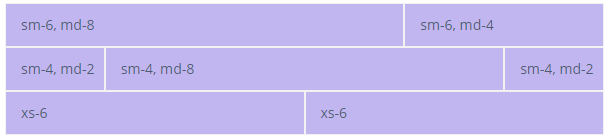As the screen narrows and width became between `576px` and `768px`, the content will look like this: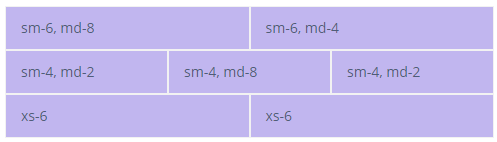And then, when the width became less than `576px`, like this: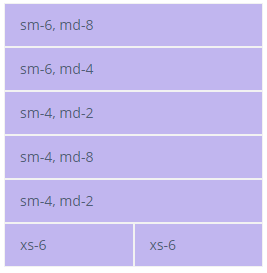For demonstration purposes, we use styles that paint over each cell: ``````@import "../helium/helium"; @mixin helium-extended { @include helium; .jmix-responsivegridlayout { .row > .col, .row > [class^=col-] { padding-top: .75rem; padding-bottom: .75rem; background-color: #c1b6ef; border: 1px solid #F2F2F2; } &.vertical-alignment { .row { min-height: 10rem; background-color: #F2F2F2; } .row + .row { margin-top: 1rem; } } } }`````` To learn more about styles, see the custom theme page.

## Col Element

### Equal-width

You can define columns without explicit values like `xs="6"`. If `cols` have no explicit values, then every column will be the same width at every breakpoint, from `xs` to `xl`. The column width, in this case, will be equal to the width of the component divided by the number of columns in the row.

For example:

``````<responsiveGridLayout>
<row>
<col>
<label value="1 of 2"/>
</col>
<col>
<label value="2 of 2"/>
</col>
</row>
<row>
<col>
<label value="1 of 3"/>
</col>
<col>
<label value="2 of 3"/>
</col>
<col>
<label value="3 of 3"/>
</col>
</row>
</responsiveGridLayout>``````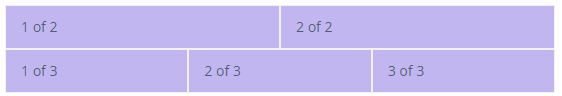### Setting One Column Width

If you specify the breakpoint value for only one column, the rest width of the container will be divided equally between the other columns.

For example:

``````<responsiveGridLayout>
<row>
<col>
<label value="1 of 3"/>
</col>
<col xs="6">
<label value="1 of 3 (wider)"/>
</col>
<col>
<label value="1 of 3"/>
</col>
</row>
<row>
<col>
<label value="1 of 3"/>
</col>
<col xs="5">
<label value="1 of 3 (wider)"/>
</col>
<col>
<label value="1 of 3"/>
</col>
</row>
</responsiveGridLayout>``````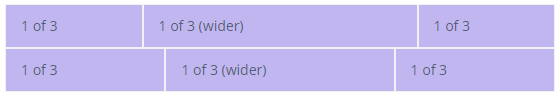### Auto and Default Width

Use `{breakpoint}="AUTO"` attributes to size columns based on the natural `width` of their content.

To create equal-width columns use `{breakpoint}="DEFAULT"`. This is the default value for the case when no explicit column value is defined.

For example:

``````<responsiveGridLayout>
<row justifyContentMd="CENTER">
<col xs="DEFAULT" lg="2">
<label value="1 of 3"/>
</col>
<col md="AUTO">
<label value="Variable width content"/>
</col>
<col xs="DEFAULT" lg="2">
<label value="1 of 3"/>
</col>
</row>
<row>
<col>
<label value="1 of 3"/>
</col>
<col md="AUTO">
<label value="Variable width content"/>
</col>
<col xs="DEFAULT" lg="2">
<label value="1 of 3"/>
</col>
</row>
</responsiveGridLayout>``````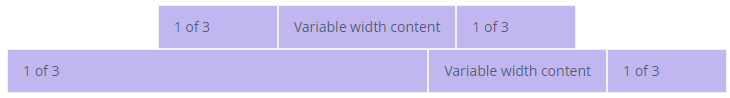## Row Element

### Cols Attributes

The following attributes define the number of columns in the `row` at a specific breakpoint:

• `cols`

• `colsLg`

• `colsMd`

• `colsSm`

• `colsXl`

For example:

``````<responsiveGridLayout stylename="demo">
<row cols="3">
<col>
<label value="Column"/>
</col>
<col>
<label value="Column"/>
</col>
<col>
<label value="Column"/>
</col>
<col>
<label value="Column"/>
</col>
</row>
</responsiveGridLayout>``````### guttersEnable attribute

By default, `guttersEnable="true"`. To disable gutters, use the `guttersEnable` attribute and set it to `false`. It removes the negative margins from the `row` and the horizontal padding from all immediate children columns.

For example:

``````<responsiveGridLayout>
<row guttersEnabled="false">
<col>
<label value="Col 1"/>
</col>
<col>
<label value="Col 2"/>
</col>
<col>
<label value="Col 3"/>
</col>
</row>
<row guttersEnabled="true">
<col>
<label value="Col 1"/>
</col>
<col>
<label value="Col 2"/>
</col>
<col>
<label value="Col 3"/>
</col>
</row>
</responsiveGridLayout>``````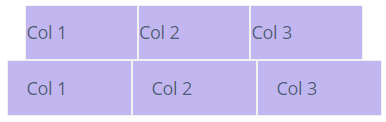## Alignment

### Vertical Alignment of Row

You can align the entire `row` vertically using the following attributes at a specific breakpoint:

• `alignItems`

• `alignItemsLg`

• `alignItemsMd`

• `alignItemsSm`

• `alignItemsXl`

For the listed above attributes, there are the following possible values:

• `BASELINE` - every `col` in a `row` is aligned as their baselines align.

• `CENTER` - every `col` in a `row` is centered.

• `END` - every `col` in a `row` is aligned to the bottom.

• `START` - every `col` in a `row` is aligned to the top.

• `STRETCH` - every `col` in a `row` is stretched to the full height of the row.

For example:

``````<responsiveGridLayout stylename="vertical-alignment">
<row alignItems="START">
<col>
<label value="1 of 3"/>
</col>
<col>
<label value="2 of 3"/>
</col>
<col>
<label value="3 of 3"/>
</col>
</row>
<row alignItems="CENTER">
<col>
<label value="1 of 3"/>
</col>
<col>
<label value="2 of 3"/>
</col>
<col>
<label value="3 of 3"/>
</col>
</row>
<row alignItems="END" >
<col>
<label value="1 of 3"/>
</col>
<col>
<label value="2 of 3"/>
</col>
<col>
<label value="3 of 3"/>
</col>
</row>
</responsiveGridLayout>``````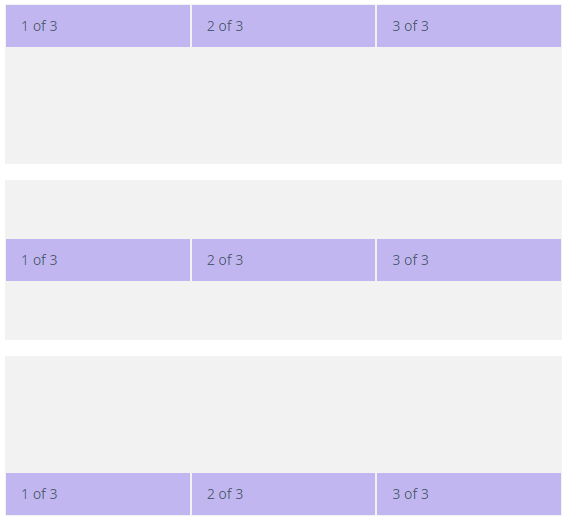### Vertical Alignment of Column

You can also align the `col` you need using the following attributes at a specific breakpoint:

• `alignSelf`

• `alignSelfLg`

• `alignSelfMd`

• `alignSelfSm`

• `alignSelfXl`

For the listed above attributes, there are the following possible values:

• `AUTO`

• `BASELINE` - `col` is aligned as its baseline is aligned.

• `CENTER` - `col` is centered.

• `END` - `col` is aligned to the bottom.

• `START` - `col` is aligned to the top.

• `STRETCH` - `col` is stretched to the full height of the row.

For example:

``````<responsiveGridLayout stylename="vertical-alignment">
<row cols="3">
<col alignSelf="AUTO">
<label value="1 of 6"/>
</col>
<col alignSelf="BASELINE">
<label value="2 of 6"/>
</col>
<col alignSelf="CENTER">
<label value="3 of 6"/>
</col>
<col alignSelf="END">
<label value="4 of 6"/>
</col>
<col alignSelf="START">
<label value="5 of 6"/>
</col>
<col alignSelf="STRETCH">
<label value="6 of 6"/>
</col>
</row>
</responsiveGridLayout>``````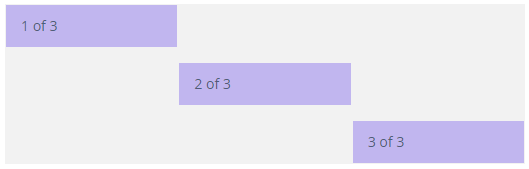### Horizontal Alignment

For horizontal alignment, you can use following attributes at a specific breakpoint:

• `justifyContent`

• `justifyContentLg`

• `justifyContentMd`

• `justifyContentSm`

• `justifyContentXl`

For the listed above attributes, there are the following possible values:

• `AROUND` - there are indents on the left, right, and between the `cols`.

• `BETWEEN` - there are indents between the `cols`.

• `CENTER` - `cols` inside the `row` are centered.

• `END` - `cols` inside the `row` aligned to the right.

• `START` - `cols` inside the `row` aligned to the left.

For example:

``````<responsiveGridLayout>
<row justifyContent="START">
<col xs="4">
<label value="1 of 2"/>
</col>
<col xs="4">
<label value="2 of 2"/>
</col>
</row>
<row justifyContent="CENTER">
<col xs="4">
<label value="1 of 2"/>
</col>
<col xs="4">
<label value="2 of 2"/>
</col>
</row>
<row justifyContent="END">
<col xs="4">
<label value="1 of 2"/>
</col>
<col xs="4">
<label value="2 of 2"/>
</col>
</row>
<row justifyContent="AROUND">
<col xs="4">
<label value="1 of 2"/>
</col>
<col xs="4">
<label value="2 of 2"/>
</col>
</row>
<row justifyContent="BETWEEN">
<col xs="4">
<label value="1 of 2"/>
</col>
<col xs="4">
<label value="2 of 2"/>
</col>
</row>
</responsiveGridLayout>``````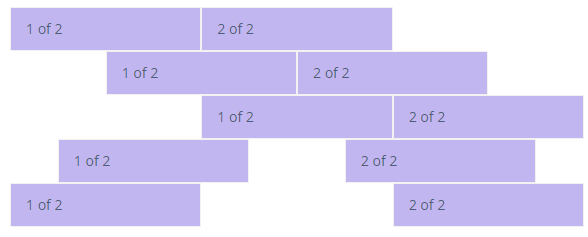## Reordering

The following attributes allow you to control the visual order of your content at a specific breakpoint:

• `order`

• `orderLg`

• `orderMd`

• `orderSm`

• `orderXl`

As a value for the listed above attributes, you can define either a particular number or one of the predefined values: `FIRST` or `LAST`.

For example:

``````<responsiveGridLayout>
<row cols="1">
<col>
<label value="First in DOM, no order applied"/>
</col>
<col order="12">
<label value="Second in DOM, with a larger order"/>
</col>
<col order="1">
<label value="Third in DOM, with an order of 1"/>
</col>
</row>
</responsiveGridLayout>

<responsiveGridLayout>
<row cols="1">
<col order="LAST">
<label value="First in DOM, ordered last"/>
</col>
<col>
<label value="Second in DOM, unordered"/>
</col>
<col order="FIRST">
<label value="Third in DOM, ordered first"/>
</col>
</row>
</responsiveGridLayout>``````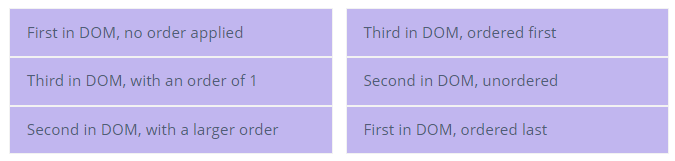## Offset

The following attributes allow you to move `cols` to the right at a specific breakpoint:

• `offset`

• `offsetLg`

• `offsetMd`

• `offsetSm`

• `offsetXl`

The offset will be calculated by multiplying the attribute value to the container’s width divided by twelve.

For example:

``````<responsiveGridLayout >
<row>
<col md="4" xl="">
<label value="md-4"/>
</col>
<col md="4" offsetMd="4">
<label value="md-4, offset-md-4"/>
</col>
</row>
<row>
<col md="3" offsetMd="3">
<label value="md-3, offset-md-3"/>
</col>
<col md="3" offsetMd="3">
<label value="md-3, offset-md-3"/>
</col>
</row>
<row>
<col md="6" offsetMd="4">
<label value="md-6, offset-md-4"/>
</col>
</row>
</responsiveGridLayout>``````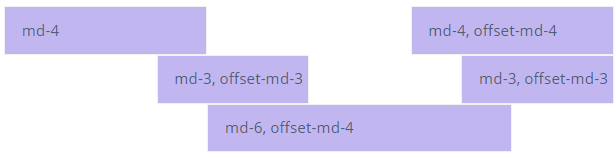## Default Bootstap Styles

You can also use default bootstrap styles via the `stylename` attribute. For example, with the `pl` value, you can set padding-left, with `mt` value, you can set margin-top, and so on. See all possible values on the bootstrap spacing page.

``````<responsiveGridLayout>
<row stylename="mt-md-n2">
<col md="AUTO" stylename="pl-0 pt-2">
<hbox spacing="true">
<label value="Col 1"/>
<textField id="nameField"/>
</hbox>
</col>
<col md="AUTO" stylename="pl-0 pt-2">
<hbox spacing="true">
<label value="Col 2"/>
<textField id="keyField"/>
</hbox>
</col>
<col md="AUTO" stylename="px-0 pt-2">
<hbox spacing="true">
<label value="Col 3"/>
<checkBox id="checkBox"/>
</hbox>
</col>
<col stylename="w-100 pl-0 pt-2">
<label value="Col 4"/>
</col>
</row>
</responsiveGridLayout>``````

## Attributes

### containerType

`ResponsiveGridLayout` has two possible types:

• `FLUID` - a full-width container that is spanning the entire width of the breakpoint. This is the default type.

• `FIXED` - once the screen width reaches a certain breakpoint, the `ResponsiveGridLayout` width is set to the following values:

1. `xl` - `1140px`

2. `lg` - `960px`

3. `md` - `720px`

4. `sm` - `540px`

For example:

``````<responsiveGridLayout containerType="FLUID">
<row>
<col sm="4">
<label value="1 of 3"/>
</col>
<col sm="4">
<label value="2 of 3"/>
</col>
<col sm="4">
<label value="3 of 3"/>
</col>
</row>
</responsiveGridLayout>

<responsiveGridLayout containerType="FIXED">
<row>
<col sm="4">
<label value="1 of 3"/>
</col>
<col sm="4">
<label value="2 of 3"/>
</col>
<col sm="4">
<label value="3 of 3"/>
</col>
</row>
</responsiveGridLayout>``````